# Strength of Materials - 1

## 20 Questions MCQ Test Mock Test Series for SSC JE Mechanical Engineering | Strength of Materials - 1

Description
Attempt Strength of Materials - 1 | 20 questions in 12 minutes | Mock test for SSC preparation | Free important questions MCQ to study Mock Test Series for SSC JE Mechanical Engineering for SSC Exam | Download free PDF with solutions
QUESTION: 1

### For the state of plane stress shown in the figure, the maximum and minimum principal stresses are: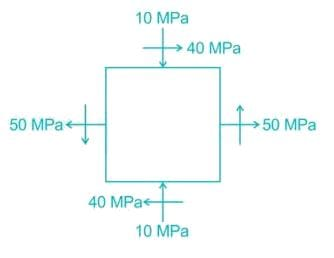Solution:

Maximum and minimum principal stresses: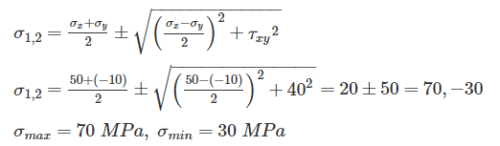QUESTION: 2

### Bending moment M and torque T is applied on a solid circular shaft. If the maximum bending stress equals to maximum shear stress developed, then M is equal to

Solution:

Bending stress due to bending moment: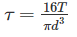Shear stress due to twisting moment/ torque: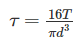If the maximum bending stress equals to maximum shear stress developed: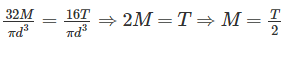QUESTION: 3

### When bending moment M and torque T is applied on a shaft then equivalent torque is

Solution:

In some applications the shaft are simultaneously subjected to bending moment M and Torque T.

From the simple bending theory equation: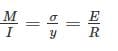If σb is the maximum bending stresses due to bending moment M on shaft: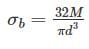Torsion equation: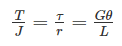The maximum shear stress developed on the surface of the shaft due to twisting moment T: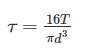Equivalent Bending Moment: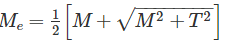Equivalent Torque: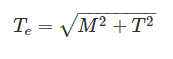QUESTION: 4

The bending moment diagram for the case shown below in Figure will be: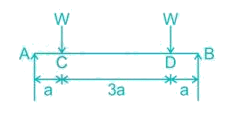Solution:

Here RA = RB = W

Between A to C:

Mx=W×x⇒Ma=0,Mc=WaMx=W×x⇒Ma=0,Mc=Wa

Between C to D:

Mx=W×x−W(x−a)⇒MD=W×4a−W(4a−a)=WaMx=W×x−W(x−a)⇒MD=W×4a−W(4a−a)=Wa

Between D to B:

Mx=W×x−W(x−a)−W(x−4a)⇒MB=W×5a−W(5a−a)−W(5a−4a)=0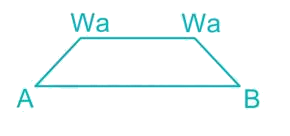QUESTION: 5

If a prismatic bar be subjected to an axial tensile stress σ, then shear stress induced on a plane inclined at θ with the axis will be

Solution:

Pn = The component of force P, normal to section FG = P cos θ

Pt = The component of force P, along the surface of the section FG (tangential to the surface FG) = P sin θ

σn = Normal Stress across the section FG

σn=σcos2θσn=σcos2θ

σt = Tangential/Shear Stress across the section FG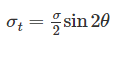QUESTION: 6

Select the proper sequence

1. Proportional Limit       2. Elastic limit

3. Yielding                      4. Failure

Solution:
•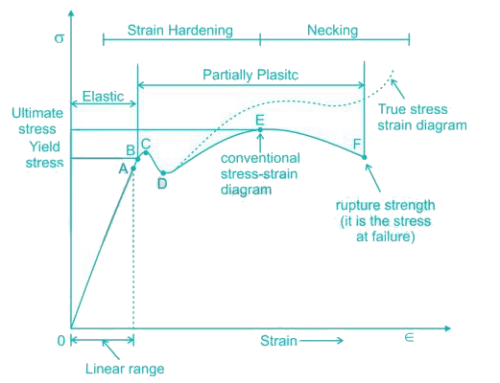• So it is evident form the graph that the strain is proportional to stress or elongation is proportional to the load giving a straight line relationship. This law of proportionality is valid upto a point A. Point A is known as the limit of proportionality or the proportionality limit.
• For a short period beyond the point A, the material may still be elastic in the sense that the deformations are completely recovered when the load is removed. The limiting point B is termed as Elastic Limit.
• Beyond the elastic limit plastic deformation occurs and strains are not totally recoverable. There will be thus permanent deformation or permanent set when load is removed. These two points are termed as upper and lower yield points respectively. The stress at the yield point is called the yield strength.
• A further increase in the load will cause marked deformation in the whole volume of the metal. The maximum load which the specimen can with stand without failure is called the load at the ultimate strength. The highest point ‘E' of the diagram corresponds to the ultimate strength of a material.
• Beyond point E, the bar begins to form neck. The load falling from the maximum until fracture occurs at F.
QUESTION: 7

In the case of bi-axial state of normal stresses, the normal stress on 45° plane is equal to

Solution:

In the case of bi-axial state of normal stresses:

Normal stress on an inclined plane: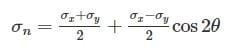Shear Stress on an inclined plane: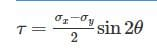Normal stress on 45° plane: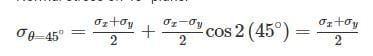QUESTION: 8

The temperature stress is a function of

1. Coefficient of linear expansion

2. Temperature rise

3. Modulus of elasticity

Solution:

Thermal strain ϵis proportional to the temperature change ΔT

ϵT=αΔTϵT=αΔT

α is coefficient of thermal expansion.

When there is some restriction to the bar to expand, thermal stress will generate in the material:

σT=strain×E=α.ΔT.E

QUESTION: 9

A steel rod of 1 sq. cm. cross sectional area is 100 cm long and has a Young’s modulus of elasticity 2 × 106 kgf/cm2. It is subjected to an axial pull of 2000 kgf. The elongation of the rod will be

Solution:

Change in length: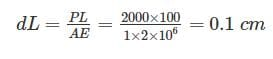QUESTION: 10

Assume that young's modulus of a wire of length L and radius 'r' is Y. If length is reduced to L/2 and radius r/2 then what will be its young's modulus?

Solution:

Young's is a property of the material of construction of a wire. It depends only on the nature of the material used for making the wire.

Young's modulus does not depend on the physical dimensions such as the length and diameter of wire. Thus there will be no change in Young's modulus.

QUESTION: 11

Circumferential and longitudinal strains in cylindrical boiler under internal steam pressure, are ε1 and ε2 respectively. Change in volume of the boiler cylinder per unit volume will be

Solution: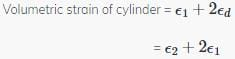QUESTION: 12

A metal pipe of 1 m diameter contains a fluid having a pressure of 10 kgf/cm2. If the permissible tensile stress in the metal is 200 kgf/cm2, then the thickness of the metal required for making the pipe would be

Solution:

As in thin cylinder shell:

Longitudinal stress = Half of circumferential stress

∴ circumferential stress ≤ Permissible stress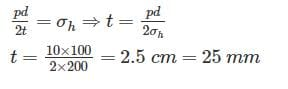QUESTION: 13

Hoops stress and longitudinal stress in a boiler shell under internal pressure are 100 MN/m2 and 50 MN/m2 respectively. Young’s modulus of elasticity and Poisson’s ratio of the shell material are 200 GN/m2 and 0.3 respectively. The hoop strain in boiler shell is

Solution: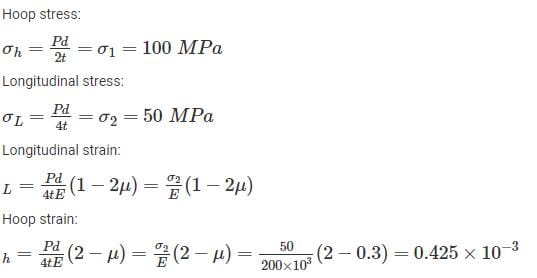QUESTION: 14

A beam cross-section is used in two different orientations as shown in figure given below: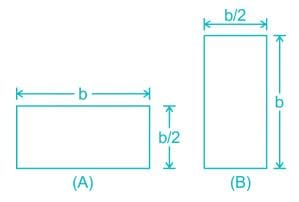Solution:

From the simple bending theory equation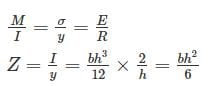If σb is the maximum bending stresses due to bending moment M on shaft: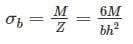For same bending moment: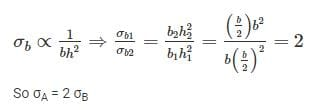QUESTION: 15

The curve ABC is the Euler’s curve for stability of column. The horizontal line DEF is the strength limit. With reference to this figure Match List-I and select the correct answer using the codes below the lines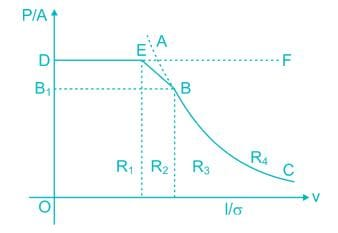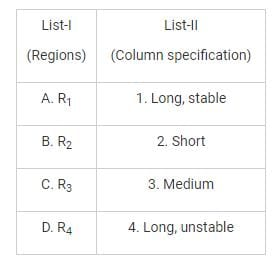Solution:

Slenderness ratio is the ratio of the length of a column and the least radius of gyration of its cross section. It is used extensively for finding out the design load as well as in classifying various columns in short/intermediate/long.

Long column: buckling occurs elastically before the yield stress is reached.

Short column: material failure occurs inelastically beyond the yield stress.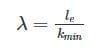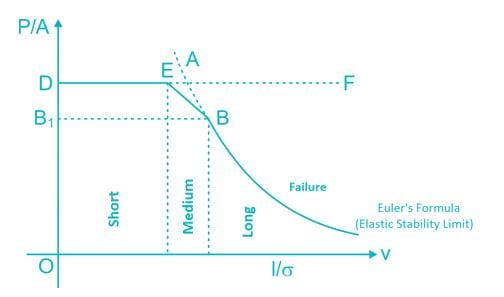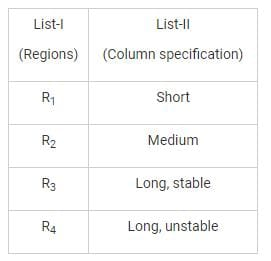QUESTION: 16

With one fixed end and other free end, a column of length L buckles at load, P1. Another column of same length and same cross-section fixed at both ends buckles at load P2. Value of P2/P1 is

Solution:

The maximum load at which the column tends to have lateral displacement or tends to buckle is known as buckling or crippling load. Load columns can be analysed with the Euler’s column formulas can be given as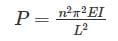• For both end hinged, n = 1
• For one end fixed and other free, n = ½
• For both end fixed, n = 2
• For one end fixed and other hinged, n = √2
•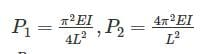•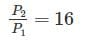QUESTION: 17

A body having weight of 1000 N is dropped form a height of 10 cm over a close-coiled helical spring of stiffness 200 N/cm. The resulting deflection of spring is nearly

Solution: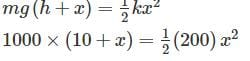100 +10x - x2 = 0

x2 -10x - 100 = 0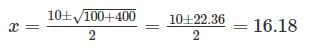x = 16 cm

QUESTION: 18

The diameter of shaft A is twice the diameter of shaft B and both are made of the same material. Assuming both the shafts to rotate at the same speed, the maximum power transmitted by B is

Solution: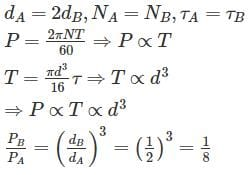QUESTION: 19

A bar having length L and uniform cross-section with area A is subjected to both tensile force P and torque T. If G is the shear modulus and E is the Young’s modulus, the internal strain energy stored in the bar is

Solution:

The elastic strain energy stored in a member of length s (it may be curved or straight) due to axial force, bending moment, shear force and torsion is summarized below: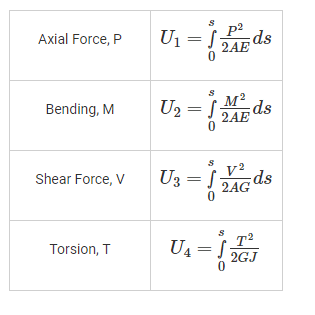Strain energy due to combined effect of load P and torque T: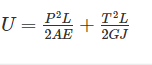QUESTION: 20

Resilience for mild steel under uni-axial tensile loading is given by the shaded portion of the stress-strain diagram as shown in

Solution:

The ability of a material to absorb energy in the elastic region. This is given by the strain energy per unit volume which is the area of the elastic region.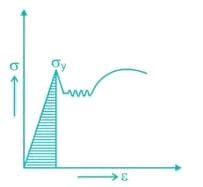Ability to absorb energy in the plastic range. This is given by the total area under the stress-strain curve.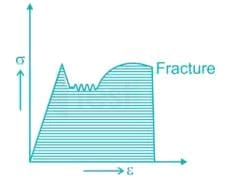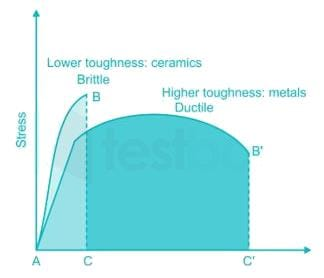Use Code STAYHOME200 and get INR 200 additional OFF Use Coupon Code